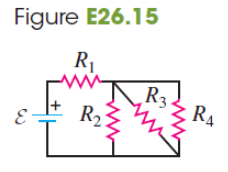# Problem: In the circuit of Fig. E26.15, each resistor represents a light bulb. Let R1 = R2 = R3 = R4 = 4.50 Ω and E = 9.00 V. (a) Find the current in each bulb. (b) Find the power dissipated in each bulb. Which bulb or bulbs glow the brightest?

###### FREE Expert Solution

Equivalent resistance for resistors in parallel:

$\overline{)\frac{\mathbf{1}}{{\mathbf{R}}_{\mathbf{eq}}}{\mathbf{=}}\frac{\mathbf{1}}{{\mathbf{R}}_{\mathbf{1}}}{\mathbf{+}}\frac{\mathbf{1}}{{\mathbf{R}}_{\mathbf{2}}}{\mathbf{+}}{\mathbf{.}}{\mathbf{.}}{\mathbf{.}}{\mathbf{+}}\frac{\mathbf{1}}{{\mathbf{R}}_{\mathbf{n}}}}$

Equivalent resistance for resistors in series:

$\overline{){{\mathbf{R}}}_{{\mathbf{eq}}}{\mathbf{=}}{{\mathbf{R}}}_{{\mathbf{1}}}{\mathbf{+}}{{\mathbf{R}}}_{{\mathbf{2}}}{\mathbf{+}}{\mathbf{.}}{\mathbf{.}}{\mathbf{.}}{\mathbf{+}}{{\mathbf{R}}}_{{\mathbf{n}}}}$

Current:

$\overline{){\mathbf{i}}{\mathbf{=}}\frac{\mathbf{V}}{\mathbf{R}}}$

Power:

$\overline{){\mathbf{P}}{\mathbf{=}}{{\mathbf{i}}}^{{\mathbf{2}}}{\mathbf{R}}}$

R2, R3, and R4 are in parallel:

1/R234 = 1/4.50 + 1/4.50 + 1/4.50 = 2/3

R234 = 1.50Ω

R1 and R234 are in series:

Req = R1234 = 4.50 + 1.50 = 6.00 Ω

88% (480 ratings)###### Problem Details

In the circuit of Fig. E26.15, each resistor represents a light bulb. Let R1 = R2 = R3 = R4 = 4.50 Ω and E = 9.00 V.(a) Find the current in each bulb.

(b) Find the power dissipated in each bulb. Which bulb or bulbs glow the brightest?